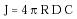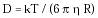Nanomedicine, Volume I: Basic Capabilities

Robert A. Freitas Jr., Nanomedicine, Volume I: Basic Capabilities, Landes Bioscience, Georgetown, TX, 1999

3.2.2 Passive Diffusive Intake

Medical nanodevices will frequently be called upon to absorb some particular material from the external aqueous operating environment. Molecular diffusion presents a fundamental limit to the speed at which this absorption can occur. (Once a block of solution has passed into the interior of a nanodevice, it may be divergently subdivided and transported at ~0.01-1 m/sec along internal pathways of characteristic dimension ~1 micron far faster than the <1 mm/sec bulk diffusion velocity across 1 micron distances; Section 9.2.7.5.)

For a spherical nanodevice of radius R, the maximum diffusive intake current is:{Eqn. 3.4}

where J is the number of molecules/sec presented to the entire surface of the device, assumed to be 100% absorbed (but see Section 4.2.5), D (m2/sec) is the translational Brownian diffusion coefficient for the molecule to be absorbed, and C (molecules/m3) is the steady-state concentration of the molecule far from the device.337 (Blood concentrations in gm/cm3 from Appendix B are converted to molecules/m3 by multiplying Appendix B figures by (106 x NA/MW), where NA = 6.023 x 1023 molecules/mole (Avogadro's number) and MW = molecular weight in gm/mole or daltons.) For rigid spherical particles of radius R, where R >> RH2O, the EinsteinStokes equation363 gives:{Eqn. 3.5}

though this is only an approximation because D varies slightly with concentration, with departure from molecular sphericalness, and other factors.

Measured diffusion coefficients in water for various molecules of physiological interest, converted to 310 K, are in Table 3.3. Diffusion coefficient data for ionic salts such as NaCl and KCl, which dissociate in water and diffuse as independent ions, are for solvated electrolytes. A 1-micron (diameter) spherical nanodevice suspended in arterial blood plasma at 310 K, with C = 7.3 x 1022 molecules/m3 of oxygen and D = 2.0 x 10-9 m2/sec, encounters a flow rate of J = 9.2 x 108 molecules/sec of O2 impinging upon its surface. (The same calculation applied to serum glucose yields J = 1.3 x 1010 molecules/sec.) The characteristic time for change mediated by diffusion in a region of size L scales as ~L2/D (Eqn. 3.9, below). Across the diameter of an L = 1 micron nanodevice, small molecules such as glucose diffuse in ~0.001 sec, small proteins like hemoglobin in ~0.01 sec, and virus particles diffuse in ~0.1 sec. Diffusion coefficients of the same molecules in air at room temperature are a factor of ~60 higher, because hair ~ 183 micropoise at 20°C.

In blood, the diffusivity of larger particles is significantly elevated because local fluid motions generated by individual red cell rotation lead to greater random excursions of the particles.388 The effective diffusivity De = D + Dr, where the rotation-induced increase in diffusivity Dr ~ 0.25 Rrbc2 'g, with red cell radius Rrbc ~ 2.8 microns (taken for convenience as a spherical volume equivalent) and a typical blood shear rate (Section 9.4.1.1) 'g ~ 500 sec-1, giving Dr ~10-9 m2/sec in normal whole blood. The elevation of diffusivity caused by red cell stirring is just 50% for O2 molecules. However, for large proteins and viruses the effective diffusivity increases 10-100 times, and the effective diffusivity of particles the size of platelets is a factor of 10,000 higher than for Brownian molecular diffusion.

The diffusion current to the surface of a nanodevice can also be estimated for various nonspherical configurations.337 For instance, the diffusion current to both sides of an isolated thin disk of radius R is given by J = 8 R D C. The two-sided current to a square thin plate of area L2 is J = (8/p1/2) L D C. The steady-state diffusion current to an isolated cylinder of length Lc and radius R is approximated by J = 2 p Lc DC / (ln(2Lc/R) - 1 ), for Lc >> R. The diffusion current through a circular hole of radius R in an impermeable wall separating regions of concentration c1 and c2 is J = 4 R D (c1 - c2).

Last updated on 7 February 2003